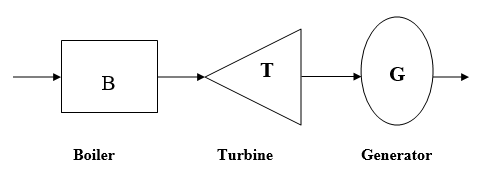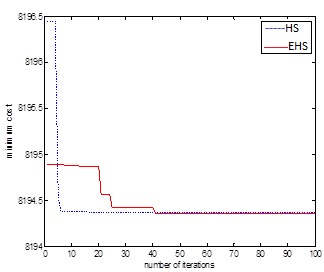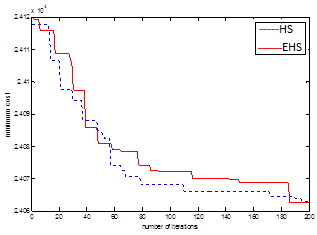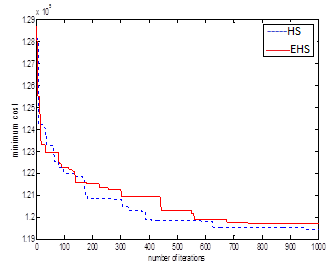# Optimal load dispatch solution of power system using enhanced harmony search algorithm

Optimal load dispatch solution of power system using enhanced harmony search algorithm

D.S.N.M. RaoNiranjan Kumar

Department of Electrical and Electronics Engineering, Vignan’s Foundation for Science, Technology, and Research, Vadlamudi, Guntur 522213, Andhra Pradesh, India

Department of Electrical and Electronics Engineering, National Institute of Technology Jamshedpur, Jharkhand 831014, India

Corresponding Author Email:
2015rsee003@nitjsr.ac.in
Page:
469-483
|
DOI:
https://doi.org/10.3166/EJEE.20.469-483
|
Accepted:
|
Published:
31 August 2018
| Citation

OPEN ACCESS

Abstract:

Keywords:

non convex, economic load dispatch, harmony search algorithm (HS), enhanced harmony search algorithm (EHS), valve point loading

1. Introduction

Operation of power system network at low price is the most precious factor and this can be achieved effectively by distributing the true power demand from available source of generation properly. Running of the system with effective economic point view termed as ED problems. The ED Problem have association to solve 2-dissimmer obstacles (Wood & Wollenberg, 1996). Here the pre dispatch obstacle is the first one that is how to meet the requirement of the load by selecting the available unit out of other units optimally for required time and online ED is second obstacle that means to decrease the generation cost how to select the one unit from parallel running units for minute to minute requirements. The effective work of ED problem is by satisfying the load demand requirement produce the real power with low price. So the production cost always depending on the load demand at particular time (Rad & Amjady, 2010).

Due to large interconnection of electrical power system, the power demand is huge and that will continuous hike in the cost of energy, so we must diminishes the operating cost of plant by minimizing the fuel consumption for a particular load. ED is one of the aspects of Optimal Power Flow (OPF) has to decrease overall input generating costs of generating units, objective function and total generation must maintain load demand and transmission line losses, equality constraints (Walters & Sheble, 1993). Traditionally ED is solved by Lagrangian approaches, Lambda-iteration approach, Newton methods, linear programming and quadratic programming and Gradient approaches (Mahor et al., 2009). For higher test systems above mentioned approaches solve the ED problem with lower efficiency.

The generation cost of the power system can be minimized with proper economic load dispatch only. To make economic dispatch as a convex issue, generating units cost function taken in quartic form this can be solved using general existed methods. By one of the conventional method we can solve the ED without considering the transmission losses (Xia & Elaiw, 2010). Another traditional approach solved ED problem by bearing in mind network transmission losses (Li & Wang, 2013), and those approaches are unable to give the exact results due to approximation power balance equations. Hence for improved solution those can be united with an iterative to obtain precise result for convex problem.

ED problem cracking approaches can be divided into three assemblies. The ﬁrst assembly comprises the approaches applied to ED problems in their unique versions. Rare samples are tabu search algorithm (TS) (Boonseng et al., 2012), particle swarm optimization (PSO), differential evolution (DE) (Iba & Noman, 2008). The approaches of the second assembly are the modiﬁed kinds of the ﬁrst assembly including modiﬁed tabu search (MTS) (Li et al., 2002), improved PSO (IPSO) (Joong-Rin et al., 2010), shufﬂed differential evolution (SDE) (Vaisakh & Srinivasa, 2013). Final assembly comprises of the mixture approaches as the grouping of approaches from the earlier groups mentioned above.

In this work, we recommend an advanced approach for cracking the ED problem by means of an Enhanced harmony search (EHS) algorithm. An ED problem constructed on a 13-unit test system (Chattopadhyay et al., 2003) through incremental fuel cost function captivating into account the valve-point loading effects is employed to validate the presentation of the EHS. The valve-point loading effects familiarize various minima in the solution space. Numerical outcomes gained with the projected EHS approach were compared with classical HS technique and other optimization outcomes stated in literature.

2. Economic load dispatch problem formulation

For newly added units in power station technical fellows got successes to enhance the output of the generating plant apparatus like boilers, generators and turbines without interruption while comparing with the older units. To decrease the cost of generation for any operating condition of power system we should know the contribution of each power station. generation of power can be done by thermal, nuclear, diesel etc. and each and every power station have their own characteristics and various cost of generations at any loads to minimize the operation cost of plant we should go with effective scheduling plants. Every generating plant cost characteristic is nonlinear (Xia et al., 2013). So to achieve the minimum operating cost also a nonlinear problem and difficult.

2.1. Cost function

The major impact on total cost of generation due to useful power only. If we want to meet more true power then we should boost up the speed of prime mover of particular plant and that will hike the fuel cost. Reactive power generation can’t affect the total cost of generation because that can be controlled by field current. Hence any generating station is for production of true power only (Jeddi & Vahidinasab, 2014).

2.1.1. Operating cost of a generator

Fuel cost, labor cost and maintains all together gives the total operating cost of generation. Generally conventional fuel power plants having low out power value that can be increased by proper adjustment of the turbine inlet valve. For amount of incoming fuel the cost of generation of all factors are fixed. Depending on the opening and closing of the turbine valve the efficiency of turbine also changes so we have open or close the valve properly depends on requirement. Fig: 1 represents the total operation involved in generation.

## 1.pngFigure 1. Thermal power generation cycle

The cost of fuel curve in true power generation has represented bellow (He et al., 2015),

${{F}_{i}}\left( {{P}_{i}} \right)={{a}_{i}}p_{i}^{2}+{{b}_{i}}{{p}_{i}}+{{c}_{i}}$Rs/hr   (1)

Here,

$F _ { i } \left( P _ { i } \right) \quad$ Generator cost function $j$

$a _ { i } , b _ { i } , c _ { i } \quad$ Generator cost coeffiecnt i

$P _ { i }$ Electrical output of generator $i ,$ MW

i set for all generators

2.1.2. Equality constrains

To reduce Objective function, Total Input Fuel Cost, power constraint must be satisfied; where the power constraint is that overall power generation should maintain overall system demand and transmission line losses.

$\sum\limits_{i=1}^{N}{{{P}_{i}}}-({{P}_{D}}+{{P}_{L}})=0$   (2)

Here, PD –overall system demand and PL- Transmission line loss. In this work PLis neglected.

2.1.3. Inequality constrains

Generated output power of each unit should be within bounds, which is satisfied the inequality constrains given by (Modiri-Delshad et al., 2016)

$P _ { \min , i } \leq P _ { i } \leq P _ { \max , i }$    (3)

Here, $P _ { \text {min} , i } , P _ { \text {max} , i }$ are the bounds of $\mathrm { f }$ it alternator.

3. Harmony search algorithm

Geem et al. (2001) suggested an effective harmony search meets-heuristic algorithm that was impressed by music process for a perfect state of harmony. Optimization technique and music in harmony both are similar and musician’s improvisations are similar to local and global quest optimization methods. Harmony Search (HS) algorithm never asks about initial values and uses a stochastic random quest instead of gradient quest that is based on Harmony Memory Considering Rate (HMCR) and the Pitch Adjusting Rate (PAR).

HS algorithm, musical performances requires an effective state of harmony find from aesthetic estimation, as the optimization algorithms requires a perfect state find from objective function value. It has been effectively applied to different optimization problems in computation and engineering sectors.

The Optimization procedure of the HS algorithm has 1-5 steps, as bellow (Javadi et al., 2012).

Step1. Frame the optimization problem, objective function with constraints and initialize the HS parameters.

Step 2. Harmony Memory (HM) is initialized.

Step 3.  New Harmony from the HM is improved.

Step 4. Modernize the memory of harmony.

Step 5. Continue the loop of steps 3 and 4 until the total iterations are completed, stopping criterion.

3.1. Enhanced harmony search (EHS) algorithm

HS is effective one to give the best performance with in minimum time, but facing problems to local quest for data applications. To enhance the effectiveness of HS algorithm and knock out the defects lie with feasible values of Harmony Memory Considering Rate (HMCR) and the Pitch Adjusting Rate (PAR). Fesanghary et al. (2007) introduced an effective HS algorithm that uses variable PAR and Band Width (BW) in improvisation step and also introduced different in harmony search, termed as global apex harmony search and main data is dumped from swarm to obtain the effective results from HS. The EHS adopted in this work have similar steps like conventional HS without step 3, and in this PAR has been dynamically changed show bellow.

$PAR=PA{{R}_{\min }}+\frac{PA{{R}_{\max }}-PA{{R}_{\min }}}{NI}*gn$   (4)

where, PAR, Pitch Adjusting Rate for generation

, PARmin is the min. adjusting rate, PARmax is the max. Adjusting rate,  is the generation number and NI is the number of solving vector generation. In addition, bandwidth for generation is dynamically updated as follows (Javadi et al., 2012).

$bw=b{{w}_{\max }}{{e}^{\left( \frac{\ln \left( \frac{b{{w}_{\min }}}{b{{w}_{\max }}} \right)}{NI}*gn \right)}}$ (5)

where,

$b w$ is the generation bandwidth,

$b w _ { \min }$ is the min. bandwidth and $b w _ { \max }$ is the max. bandwidth

3.2. Application of harmony search algorithm to ED problem

After the initial development of HS algorithm in 2001, HS algorithm applications have been expanded in a huge range of obstacles. The scientific range is so broad, that one can conclude that Harmony Search Algorithm has generally accepted as a robust optimization technique (Coelhos & Mariani, 2009).

Step1. Initialize parameters.

Step2. Initialize the harmony memory.

Step3. Improvise a new harmony

Step5. Update harmony memory

Step6. Stopping criterion

3.3. Application of enhanced harmony search algorithm to ED problem

Although HSA has better capability in identifying the search space in sensible time it is not capable in finding local optimums in case of numerical issues. The drawbacks like fixed values of HMCR and fewer PAR values with large band widths may reduce the performance. Hence increase in iteration number may happen to find optimal solution. So 4 and 5 equations are utilized to change BW and PAR values.

4. Simulation results and discussions

The adopted algorithms HS, EHS were tested on 3- standard load dispatch problems consisting of 3-13 and 40-units and implemented by MATLAB 8.5 R2016b. Here selection of parameters in adopted method is somewhat difficult. Optimal parameter setting for the all test systems is given in Table I & Table II.

Table 1. Optimal parameter setting of HS, EHS for 3 and 13 units

 Parameters 3- Units 13- Units HS EHS HS EHS pop 30 30 130 130 HMCR 0.95 0.95 0.95 0.95 PAR 0.45 -- 0.45 -- PARmin -- 0.4 -- 0.4 PARmax -- 0.99 -- 0.99 BW 0.01 0.01 0.01 0.01 BWmin -- 0.00005 -- 0.00005 BWmax -- 0.05 -- 0.05 iter 100 100 200 200
Table 2. Optimal parameter setting of HS, EHS for 13 and 40 units
 Parameters 13- Units 40- Units HS EHS HS EHS pop 130 130 400 400 HMCR 0.95 0.95 0.95 0.95 PAR 0.45 -- 0.45 -- PARmin -- 0.4 -- 0.4 PARmax -- 0.99 -- 0.99 BW 0.01 0.01 0.01 0.01 BWmin -- 0.00005 -- 0.00005 BWmax -- 0.05 -- 0.05 iter 200 200 500 500
4.1. For three unit system

A system of 3-thermal generating units with the quadratic cost function has been considered in this test. All generating units collectively meet the total demand of 850MW. Global optimal solution for this three-unit test system is attained as 8194.356124$/hr (Xia et al., 2013). The dispatch results using the proposed methods, HS, EHS are given Table 3. From Table 3, it is evident that the low cost gained by all the methods is same as the global solution. For this test system, hundred iteration (individual trails) have been made. Based on results obtained, the comparison of HS, EHS applied to thermal units are presented in Table III. The convergence criteria of HS, EHS methods for the three unit system are shown in Fig 2. Table 3. Comparisons of simulation results for 3-unit system  Unit Method HS EHS G1 330.344330 443.285943 G2 399.090808 281.588517 G3 120.564862 125.125540 Minimum cost ($/h) 8194.368755 8194.356124 Total power (MW) 850 850

## 2.pngFigure 2. Convergence criterion for 3-unit system

4.2. For thirteen unit system

Here, the proposed methods are applied to the 13-generator unit test system that includes quadratic generator input cost functions. Two dissimilar power demands have been used to show the efficacy of adopted algorithms in obtaining optimum solutions. Two different case studies have been considered on this test system. At first a load demand of 1800MW is taken (Coelhos & Mariani, 2009) and later a load demand of 2520MW is considered.

Convergence criteria and the output power generations (MW) of HS and EHS methods applied to thirteen unit test system operating on a load demand of 1800MW is shown in Fig 3 and Table 4 respectively.  From Table 4, it is clear that the low price gained from adopted approach is 17935.683284$/hr. Here we got the results by all the methods is same as the global solution. Table 4. Comparisons of simulation results for 13-unit system PD=1800 MW  Unit Method HS EHS G1 623.150720 494.415492 G2 19.859718 126.920922 G3 195.827057 352.627500 G4 73.273771 73.556964 G5 171.449723 156.961100 G6 118.821822 68.377544 G7 75.778269 61.632842 G8 108.008892 121.345118 G9 99.879446 66.173336 G10 74.288292 68.534340 G11 87.001353 40.644621 G12 63.080884 100.643458 G13 89.580050 68.1 66764 Minimum Cost($/hr) 17935.418707 17935.683284 Total Power (MW) 1800 1800

Table 5. Comparisons of simulation results for 13-unit system PD=2520 MW

 Unit Method HS EHS G1 680.000000 680.000000 G2 277.380097 209.329820 G3 357.803149 314.965502 G4 101.803996 205.115220 G5 125.653425 203.759629 G6 161.343304 94.586705 G7 134.837412 118.625349 G8 151.287822 144.707589 G9 104.156086 105.136503 G10 117.409011 76.195748 G11 93.878199 133.645069 G12 115.492372 126.241200 G13 98.955126 107.691666 Minimum cost($/hr) 24062.989996 24062.580327 Total Power (MW) 2520 2520 ## 3.pngFigure 3. Convergence criterion for 13-unit system with PD=1800 MW ## 4.pngFigure 4. Convergence criterion for 13-unit system with PD=2520MW Table 5. shows that dispatch solutions HS, EHS methods to a load of 2520MW, Output gained from all methods, it is evident that the power balance constraint is compromised after sixth decimal also. Result obtained by proposed approach is compared with the HS, EHS as presented in Table 5. The least price gotten by the adopted approach is 24062.580327$/hr. This can be achieved by balancing the power constraints. Fig 4 shows illustrate the convergence criterion of proposed algorithms for the 13-unit system with a load demand of 2520 MW.

4.3. For forty unit system

Table 6. Simulation results of HS and EHS methods for 40-unit system

 Unit Method HS EHS G1 113.999232 113.999014 G2 114.000000 114.000000 G3 120.000000 120.000000 G4 190.000000 190.000000 G5 97.000000 97.000000 G6 140.000000 140.000000 G7 300.000000 300.000000 G8 300.000000 300.000000 G9 300.000000 300.000000 G10 130.000000 130.000000 G11 94.000000 94.000000 G12 94.000000 94.000000 G13 125.000000 125.000000 G14 271.853430 270.842149 G15 265.890514 266.432925 G16 267.256825 267.726011 G17 500.000000 500.000000 G18 500.000000 500.000000 G19 550.000000 550.000000 G20 550.000000 550.000000 G21 550.000000 550.000000 G22 550.000000 550.000000 G23 550.000000 550.000000 G24 550.000000 550.000000 G25 550.000000 549.999900 G26 550.000000 550.000000 G27 10.000000 10.000000 G28 10.000000 10.000000 G29 10.000000 10.000000 G30 97.000000 97.000000 G31 190.000000 190.000000 G32 190.000000 190.000000 G33 190.000000 190.000000 G34 200.000000 200.000000 G35 200.000000 200.000000 G36 200.000000 200.000000 G37 110.000000 110.000000 G38 110.000000 110.000000 G39 110.000000 110.000000 G40 550.000000 550.000000 Minimum Cost ($/hr.) 119450.643035 118660.253435 Total Power (MW) 10500 10500 Here adopted methods are implanted to forty thermal units with the quadratic input fuel cost function. Here the load demand expected to be met by generating units is 10500 MW. Result obtained by implemented approach with 100 trails, on the 40 thermal units (Li & Wang, 2013) test is given in Table 6. It can be cleared that the adopted approach achieved in obtaining believable result of 40-unit test system & has represented the importance to the other approaches for 40-unit systems. The final low cost gained by the adopted approach for 40- unit system is 119441.588684$/hr. Fig 5 shows illustrate the convergence criterion of proposed algorithms for the 40-unit system with a load demand of 10500 MW.

## 5.pngFigure 5. Convergence criterion for 40-unit system with PD=10500 MW

5. Conclusion

In this work heuristic approach such as Harmony Search and Enhanced Harmony Search algorithms effectively implemented to solve power system ED problem. The usefulness, perfectness of the adopted algorithm tested on electrical network having three, thirteen and forty units. From three systems simulation results we overcome the problem with reduction in cost of generation. Adopted method highly increased the searching capability and perfectly handled the system constraints. The successful global optimizing performance on the validation data set shows the efficiency of the adopted approach and show that it can be used as a dependable tool for solving ED problem.

References

Boonseng C., Khamsawang S., Pothiya S. (2012). Solving the economic dispatch problem with tabu search algorithm. IEEE Conference on ICIT, Bangkok, Vol. 1, pp. 274-278. https://doi.org/10.1109/ICIT.2002.1189906

Chattopadhyay P. K., Sinha N., Chakrabarti R. (2003). Evolutionary programming techniques for economic load dispatch. IEEE Transactions on Evolutionary Computation, Vol. 7, No. 1, pp. 83–94. https://doi.org/10.1109/TEVC.2002.806788

Coelhos L. D. S., Mariani V. C. (2009). An improved harmony search algorithm for power economic load dispatch. Energy Conversion and Management, Vol. 50, pp. 2522–2526. https://doi.org/10.1016/j.enconman.2009.05.034

Fesanghary M., Damangir E., Mahdavi M. (2007). An improved harmony search algorithm for solving optimization problems. Applied Mathematics and Computation, Vol. 188, pp. 1567-1579. https://doi.org/10.1016/j.amc.2006.11.033

Geem Z. W., Kim J. H., Loganathan G. V. (2001). A new heuristic optimization algorithm: Harmony search. Simulation, Vol. 76, No. 2, pp. 60-68. https://doi.org/10.1177/003754970107600201

He X. Z., Rao Y. Q., Huang J. D. (2015). A novel algorithm for economic load dispatch of power systems. Neurocomputing, Vol. S0925-2312, No. 15, 01156-X. https://doi.org/10.1016/j.neucom.2015.07.107

Iba H., Noman N. (2008). Differential evolution for economic load dispatch problems. Electric Power Systems Research, Vol. 78, No. 8, pp. 1322-31. https://doi.org/10.1016/j.epsr.2007.11.007

Javadi M. S., Nezhad A. E., Sabramooz S. (2012). Economic heat and power dispatch in modern power system harmony search algorithm versus analytical solution. Scientia Iranica D, Vol. 19, No. 6, pp. 1820–1828. https://doi.org/10.1016/j.scient.2012.10.033

Jeddi B., Vahidinasab V. (2014). A modified harmony search method for environmental/economic load dispatch of real-world power systems. Energy Conversion and Management, Vol. 78, pp. 661–675. https://doi.org/10.1016/j.enconman.2013.11.027

Joong-Rin S, Yun-Won J, Lee K. Y., Jong-Bae P. (2010). An improved particle swarm optimization for nonconvex economic dispatch problems. IEEE Transactions on Power Systems, Vol. 25, No. 1, pp.156-166. https://doi.org/10.1109/TPWRS.2010.2069870

Li L. P., Wang L. (2013). An effective differential harmony search algorithm for the solving non-convex economic load dispatch problems. Electrical Power and Energy Systems, Vol. 44, pp. 832–843. https://doi.org/10.1016/j.ijepes.2012.08.021

Lin W. M., Chen F. S., Tsay M. T. (2002). An improved tabu search for economic dispatch with multiple minima. IEEE Transactions on Power Systems, Vol. 17, No. 1, pp. 108-112. https://doi.org/10.1109/mper.2002.4311692

Mahor A., Rangnekar S., Prasad V. (2009). Economic dispatch using particle swarm optimization: A review. Renewable and Sustainable Energy Reviews, Vol. 13, pp. 2134–2141. https://doi.org/10.1016/j.rser.2009.03.007

Modiri-Delshad M., Hr S., Kaboli A., Taslimi-Renani E., Rahim N. A. (2016). Backtracking search algorithm for solving economic dispatch problems with valve-point effects and multiple fuel options. Energy, Vol. 116, pp. 637-649. https://doi.org/10.1016/j.energy.2016.09.140

Rad H. N., Amjady N. (2010). Solution of nonconvex and nonsmooth economic dispatch by a new adaptive real coded genetic algorithm. Expert Systems with Applications, Vol. 37, pp. 5239–5245. https://doi.org/10.1016/j.eswa.2009.12.084

Vaisakh K., Srinivasa R. A. (2013). Shuffled differential evolution for large scale economic dispatch. Electric Power Systems Research, Vol. 96, pp. 237-245. https://doi.org/10.1016/j.ijepes.2012.10.012

Walters D. C., Sheble G. B. (1993). Genetic algorithm solution of economic dispatch with valve point loading. IEEE Transactions on Power Systems, Vol. 8, No. 3, pp. 1325-1332. https://doi.org/ 10.1109/59.260861

Wood A. J., Wollenberg B. F. (1996). Power generation, operation, and control. Second Edition, A Wiley Inter science Publication, New York. https://trove.nla.gov.au/work/21096119

Xia H. G., Chen D. L., Gao L. Q. (2013). Modified harmony search algorithm for power economic load dispatch. Journal of Computational Information Systems, Vol. 9, No. 5, pp. 2103–2110. https://doi.org/10.4028/www.scientific.net/AMR.989-994.2528

Xia X., Elaiw A. M. (2010). Optimal dynamic economic dispatch of generation: A review. Electric Power Systems Research, Vol. 80, pp. 975–986. https://doi.org/10.1016/j.epsr.2009.12.012